# Test: Scalar Product Of Two Vectors

## 5 Questions MCQ Test Mathematics (Maths) Class 12 | Test: Scalar Product Of Two Vectors

Description
This mock test of Test: Scalar Product Of Two Vectors for JEE helps you for every JEE entrance exam. This contains 5 Multiple Choice Questions for JEE Test: Scalar Product Of Two Vectors (mcq) to study with solutions a complete question bank. The solved questions answers in this Test: Scalar Product Of Two Vectors quiz give you a good mix of easy questions and tough questions. JEE students definitely take this Test: Scalar Product Of Two Vectors exercise for a better result in the exam. You can find other Test: Scalar Product Of Two Vectors extra questions, long questions & short questions for JEE on EduRev as well by searching above.
QUESTION: 1

### If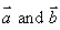are any two vectors, then

Solution:

|a+b| ≤ |a| + |b|
Let us take an example : a = 1, b= 2
|1 + 2| ≤ |1| + |2|
|3| ≤ |3|
Hence, proved

QUESTION: 2

### The angle between the vectors is: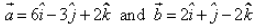is :

Solution:

a = 6i - 3j + 2k      b = 2i + j - 2k
a.b = 12 - 3 - 4 = 5
|a| = [(6)2 + (-3)2 + (2)2]1/2
|a| = [36 + 9 + 4]½
|a| = (49)½
|a| = 7
|b| = [(2)2 + (1)2 + (-2)2]½
|b| = [4 + 1 + 4]½
|b| = 3
Cosθ = (a.b)/|a||b|
= 5/(7)(3)
= 5/21
θ = cos-1(5/21)

QUESTION: 3

### Ifare two vectors, such that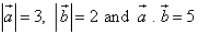, then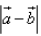= ……​

Solution:

|a - b|2 = |a|2 + |b|2 - 2|a||b|
|a - b|2  = (3)2 + (2)2 - 2(5)
|a - b|2  = 9 + 4 - 10
|a - b|2  = 3
|a - b|   = (3)½.

QUESTION: 4

The projection of the vector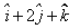on the vector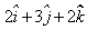is:​

Solution:

Projection = (A.B)/|B|
= [(i + 2j + k) . (2i + 3j + 2k)]/[(2)2 + (3)2 + (2)2]½
= (2 + 6 + 2)/[4 + 9 + 4]½
= 10/(17)1/2

QUESTION: 5

The angle between two non-zero vectors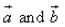is given by

Solution:

A sequence is a function whose domain is the set of natural numbers or a subset of the natural numbers. We usually use the symbol an to represent a sequence, where n is a natural number and an is the value of the function on n. A sequence may be finite or infinite.